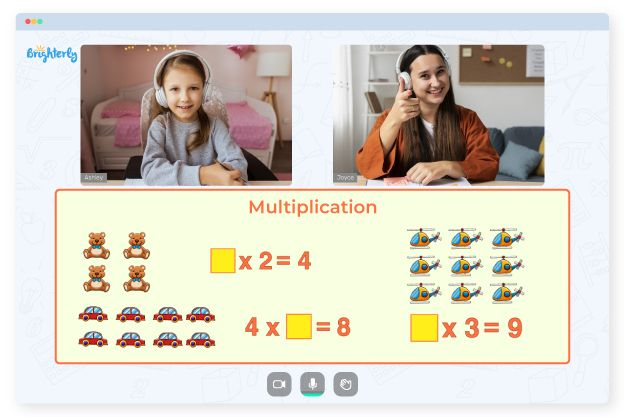As children progress in their foundational classes, they learn more complicated math concepts. Multiplication is one of such topics, and you need extra help to make sure your kids understand it. With multiplication worksheets, grade 2 students can learn multiplication faster, and the benefits don’t end there.

## Benefits of multiplication worksheets for grade 2

Grade 2 students are still trying to understand the actual life application of math skills. Showing them that multiplication could increase a figure by far or rather rapidly may confuse them. Using 2nd grade multiplication worksheets helps them practice multiplication at home and better understand it.

## Types of second grade multiplication worksheets

The typical way for kids to learn multiplication is by using tables. But there are different types of multiplication worksheets 2nd graders can also benefit from. Each has specific methods, so it’s your job to figure out what type of worksheets your students are comfortable with and capitalize on the usage.

Math for Kids

Is Your Child Struggling With Math?
1:1 Online Math Tutoring### Printable multiplication worksheets

You can download printable multiplication worksheets off the internet, and they come in different styles. These worksheets teach the children multiplication using rapid addition and counting methods. Download them and share them with your kids.

### Interactive multiplication worksheets

Interactive worksheets help you see how many students understand what you are teaching. These are worksheets that encourage peer-to-peer learning.### Multiplication Worksheets Grade 2 PDF### Multiplication Worksheets Grade 2 PDF### Multiplication Worksheets Grade 2 PDF### Multiplication Worksheets Grade 2 PDF

Interactive worksheets reduce your work as a teacher by a small percentage and help you see how well your students have learned. Instead of taking the sheets home to practice, students can practice independently, improving understanding. Children can sit in circles and ask questions from their worksheets while trying to understand what the other person’s worksheet is teaching.

### More Multiplication Worksheets

Struggling with Multiplication?• Is your child finding multiplication concepts challenging?
• An online tutor could provide the necessary assistance.

Does your child struggle with understanding the concept of multiplication? Try lessons with an online tutor.